# Synchronous Sequential Circuits in Digital Logic

Synchronous sequential circuits are digital circuits that use clock signals to determine the timing of their operations. They are commonly used in digital systems to implement timers, counters, and memory elements.

1. In a synchronous sequential circuit, the state of the circuit changes only on the rising or falling edge of the clock signal, and all changes in the circuit are synchronized to this clock. This makes the behavior of the circuit predictable and ensures that all elements of the circuit change at the same time, preventing race conditions and making the circuit easier to design and debug.
2. Synchronous sequential circuits can be implemented using flip-flops, which are circuits that store binary values and maintain their state even when the inputs change. The output of the flip-flops is determined by the current inputs and the previous state stored in the flip-flops, and the next state is determined by the state transition function, which is a Boolean function that describes the behavior of the circuit.
3. In summary, synchronous sequential circuits are digital circuits that use clock signals to determine the timing of their operations. They are commonly used in digital systems to implement timers, counters, and memory elements and are essential components in digital systems design.

Steps to solve a problem:
1. Draw the state diagram from the problem statement or from the given state table. Example: Serial Adder. The functioning of serial adder can be depicted by the following state diagram. X1 and X2 are inputs, A and B are states representing carry.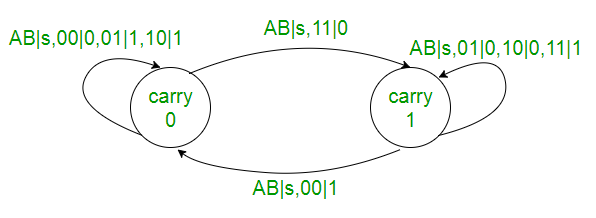2. Draw the state table. If there is any redundant state then reduce the state table.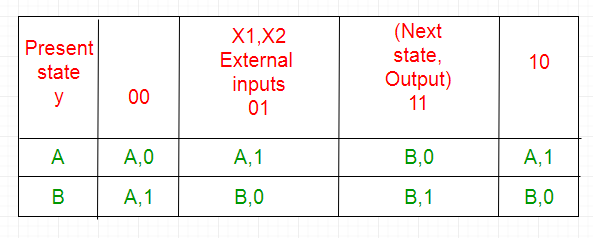3. Select state assignment i.e. assign binary numbers to the states according to total number states. Also decide the memory element (flip-flops) for the circuit. A -> 0 B -> 1 4. Replace the assignments in the state table to obtain Transition table: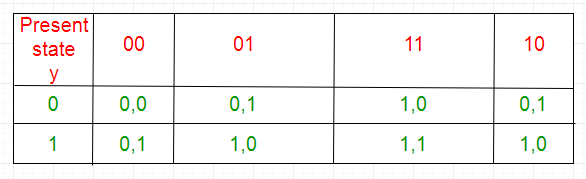5. Separate the output table from the transition table.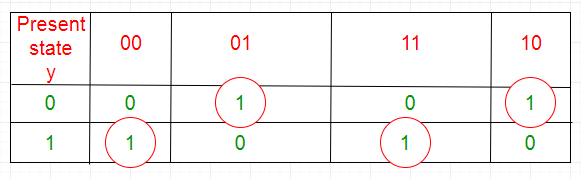z = x1x’2y+x’1x2y’+x1x2y+x1x’2y’

6. Excitation table for the flip-flop is obtained from the transition table using the output of flip-flop. Excitation table for D flip-flop: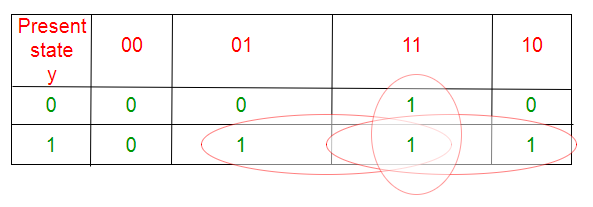D = x1 x2 +x1 y+x2 y

7. Draw the circuit diagram using gates and flip-flops.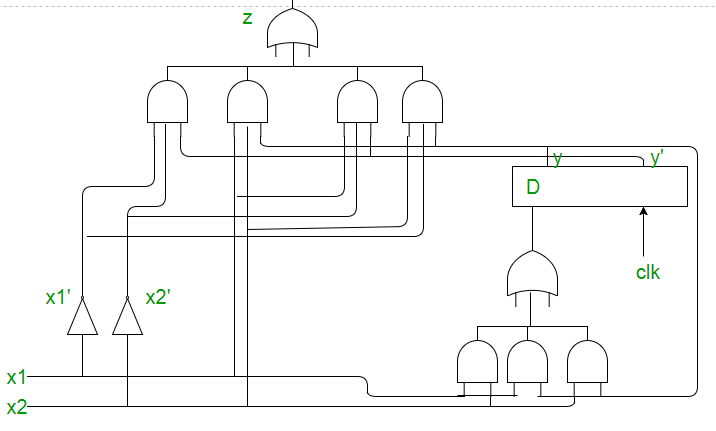### Advantages of Synchronous Sequential Circuits:

1. Predictable behavior: The use of a clock signal makes the behavior of a synchronous sequential circuit predictable and deterministic, which is important for real-time control applications.
2. Synchronization: Synchronous sequential circuits ensure that all elements of the circuit change at the same time, preventing race conditions and making the circuit easier to design and debug.
3. Timing constraints: The timing constraints in a synchronous sequential circuit are well-defined, making it easier to design and test the circuit.
4. Easy to implement: Synchronous sequential circuits can be implemented using flip-flops, which are simple and widely available digital components.

### Disadvantages of Synchronous Sequential Circuits:

1. Clock skew: Clock skew is a timing error that occurs when the clock signal arrives at different flip-flops at different times. This can cause errors in the operation of the circuit.
2. Timing jitter: Timing jitter is a variation in the arrival time of the clock signal that can cause errors in the operation of the circuit.
3. Complex design: The design of synchronous sequential circuits can be complex, especially for large systems with many state transitions.
4. Power consumption: The use of a clock signal increases the power consumption of a synchronous sequential circuit compared to asynchronous sequential circuits.

### REFERENCE:

Here are a few books that you can refer to for further information on synchronous sequential circuits:

1. “Digital Systems Design Using VHDL” by Charles H. Roth Jr. and Lizy Kurian John
2. “Digital Circuit Design: An Introduction” by Thomas L. Floyd and David Money Harris
3. “Digital Design and Computer Architecture” by David Harris and Sarah Harris
4. “Principles of Digital Design” by Daniel D. Gajski, Frank Vahid and Tony Givargis
5. “Digital Design: Principles and Practices” by John F. Wakerly

These books cover various topics in digital logic and design, including synchronous sequential circuits, and provide in-depth information on the theory, design, and implementation of digital circuits.

Whether you're preparing for your first job interview or aiming to upskill in this ever-evolving tech landscape, GeeksforGeeks Courses are your key to success. We provide top-quality content at affordable prices, all geared towards accelerating your growth in a time-bound manner. Join the millions we've already empowered, and we're here to do the same for you. Don't miss out - check it out now!

Previous
Next